# 3 Structures and Strategies for State Space Search

• Slides: 55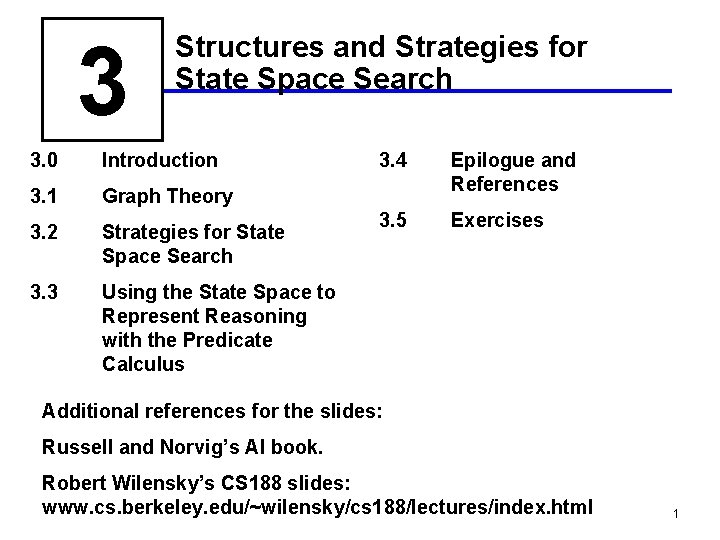3 Structures and Strategies for State Space Search 3. 0 Introduction 3. 1 Graph Theory 3. 2 Strategies for State Space Search 3. 3 Using the State Space to Represent Reasoning with the Predicate Calculus 3. 4 Epilogue and References 3. 5 Exercises Additional references for the slides: Russell and Norvig’s AI book. Robert Wilensky’s CS 188 slides: www. cs. berkeley. edu/~wilensky/cs 188/lectures/index. html 1Chapter Objectives • Learn the basics of state space representation • Learn the basics of search in state space • The agent model: Has a problem, searches for a solution. 2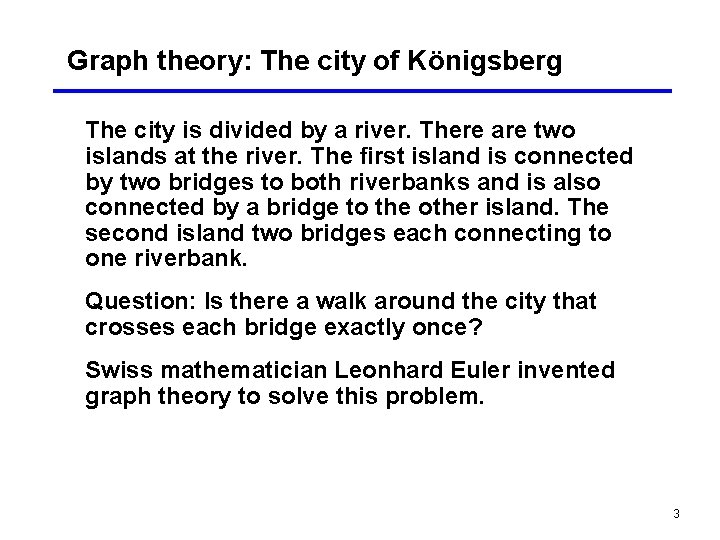Graph theory: The city of Königsberg The city is divided by a river. There are two islands at the river. The first island is connected by two bridges to both riverbanks and is also connected by a bridge to the other island. The second island two bridges each connecting to one riverbank. Question: Is there a walk around the city that crosses each bridge exactly once? Swiss mathematician Leonhard Euler invented graph theory to solve this problem. 3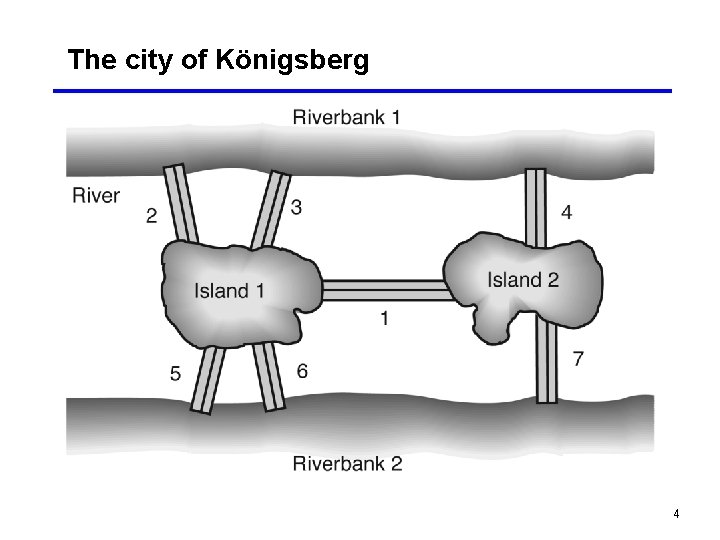The city of Königsberg 4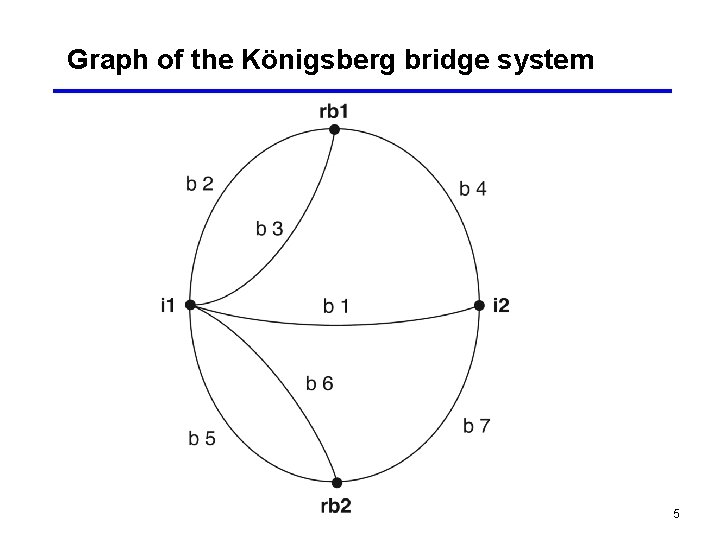Graph of the Königsberg bridge system 5Definition of a graph A graph consists of • A set of nodes (can be infinite) • A set of arcs that connect pairs of nodes. An arc is an ordered pair, e. g. , (i 1, rb 1), (rb 1, i 1). 6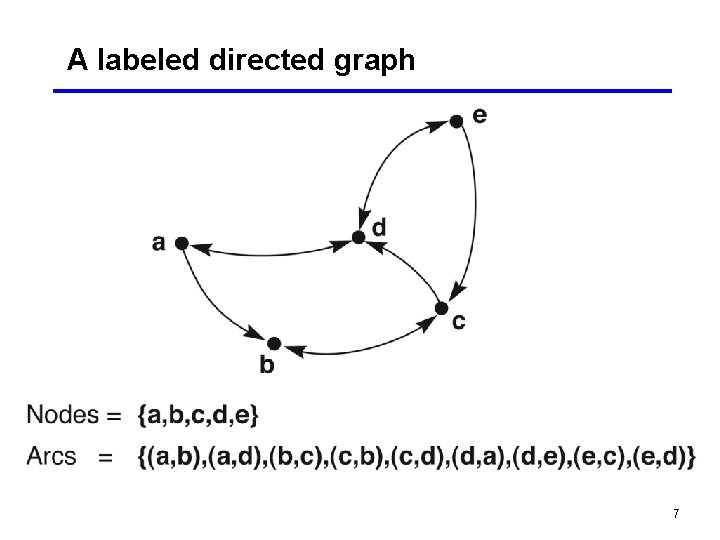A labeled directed graph 7A rooted tree, exemplifying family relationships 8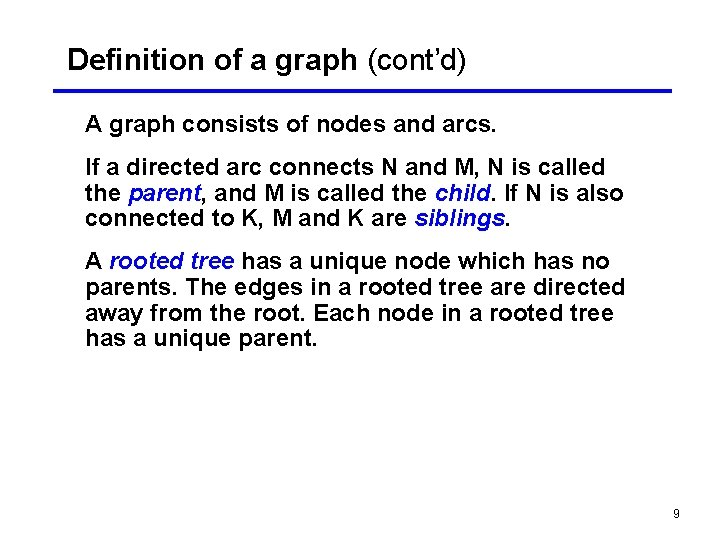Definition of a graph (cont’d) A graph consists of nodes and arcs. If a directed arc connects N and M, N is called the parent, and M is called the child. If N is also connected to K, M and K are siblings. A rooted tree has a unique node which has no parents. The edges in a rooted tree are directed away from the root. Each node in a rooted tree has a unique parent. 9Definition of a graph (cont’d) A leaf or tip node is a node that has no children (sometimes also called a dead end). A path of length n is an ordered sequence of n+1 nodes such that the graph contains arcs from each node to the following ones. E. g. , [a b e] is a path of length 2. On a path in a rooted graph, a node is said to be an ancestor of all the nodes positioned after it (to its right), as well as a descendant of all nodes before it (to its left). 10Definition of a graph (cont’d) A path that contains any node more than once is said to contain a cycle or loop. A tree is a graph in which there is a unique path between every pair of nodes. Two nodes are said to be connected if a path exists that includes them both. 11A unifying view (Newell and Simon) The problem space consists of: • a state space which is a set of states representing the possible configurations of the world • a set of operators which can change one state into another The problem space can be viewed as a graph where the states are the nodes and the arcs represent the operators. 12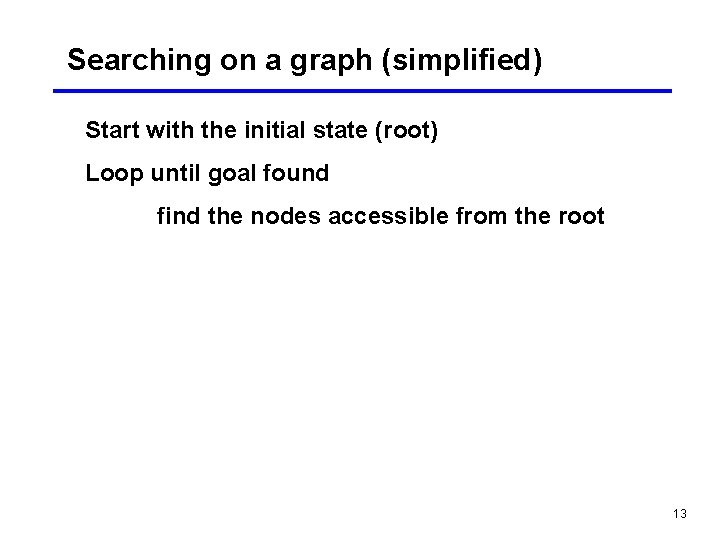Searching on a graph (simplified) Start with the initial state (root) Loop until goal found find the nodes accessible from the root 13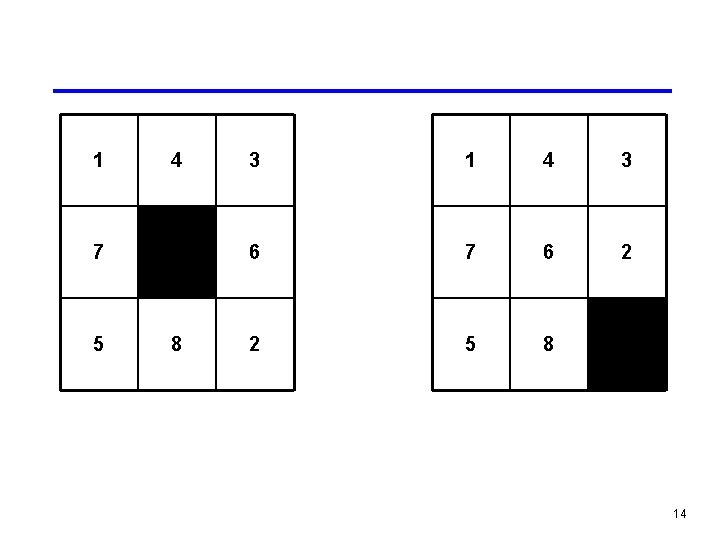1 4 7 5 8 3 1 4 3 6 7 6 2 2 5 8 14State space of the 8 -puzzle generated by “move blank” operations 15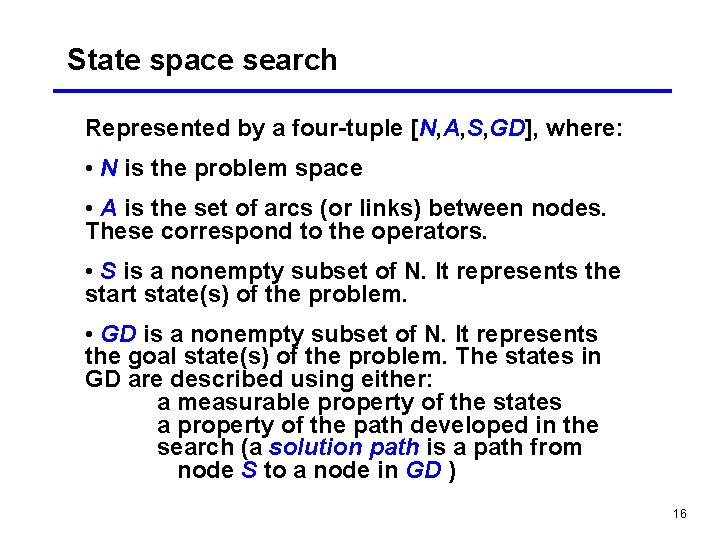State space search Represented by a four-tuple [N, A, S, GD], where: • N is the problem space • A is the set of arcs (or links) between nodes. These correspond to the operators. • S is a nonempty subset of N. It represents the start state(s) of the problem. • GD is a nonempty subset of N. It represents the goal state(s) of the problem. The states in GD are described using either: a measurable property of the states a property of the path developed in the search (a solution path is a path from node S to a node in GD ) 16The 8 -puzzle problem as state space search • states: possible board positions • operators: one for sliding each square in each of four directions, or, better, one for moving the blank square in each of four directions • initial state: some given board position • goal state: some given board position Note: the “solution” is not interesting here, we need the path. 17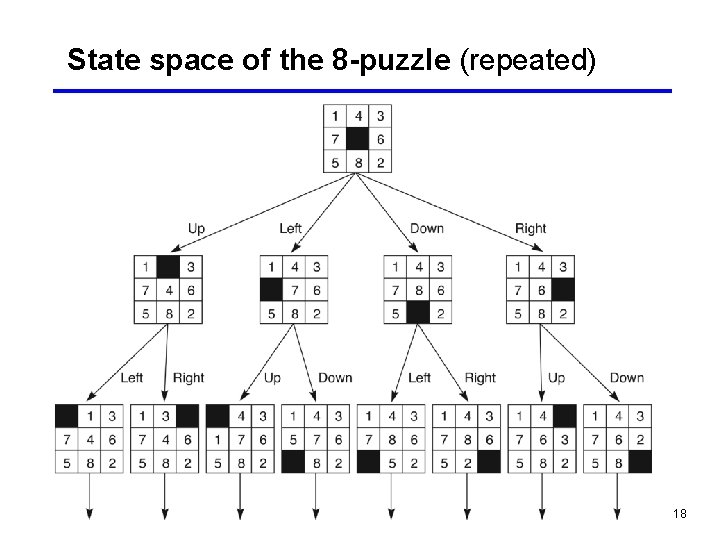State space of the 8 -puzzle (repeated) 18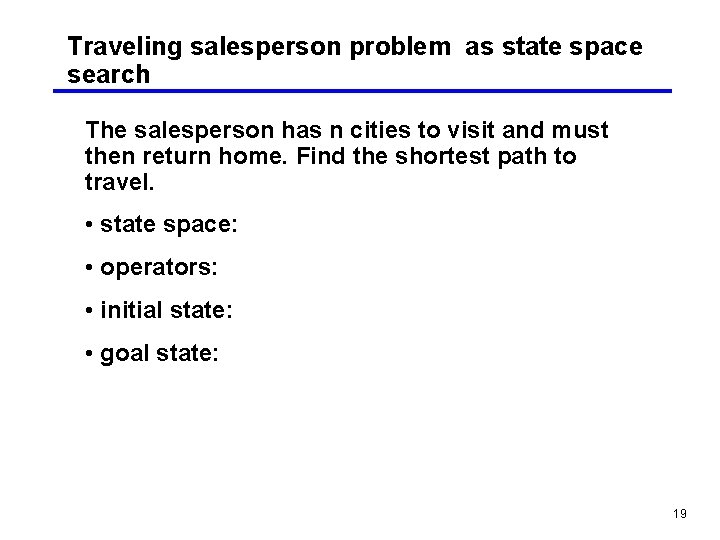Traveling salesperson problem as state space search The salesperson has n cities to visit and must then return home. Find the shortest path to travel. • state space: • operators: • initial state: • goal state: 19An instance of the traveling salesperson problem 20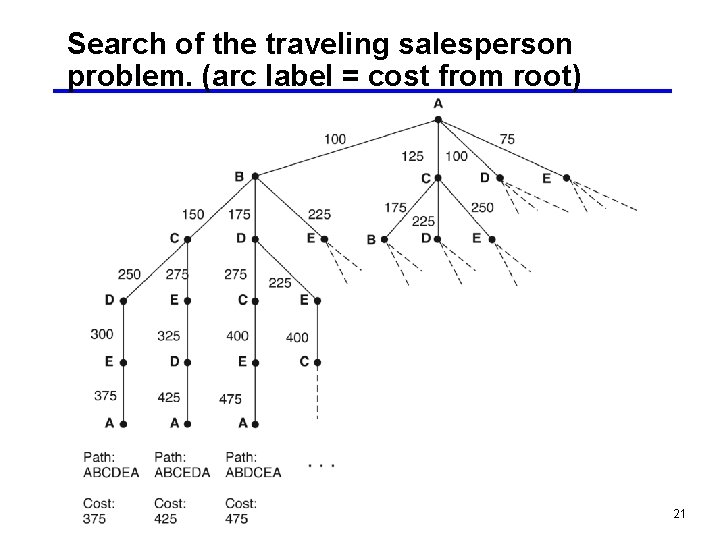Search of the traveling salesperson problem. (arc label = cost from root) 21Nearest neighbor path = AEDBCA (550) Minimal cost path = ABCDEA (375) 22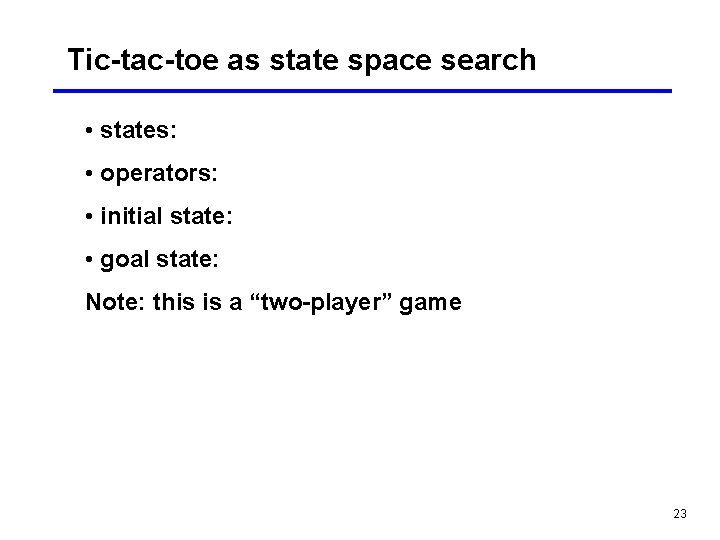Tic-tac-toe as state space search • states: • operators: • initial state: • goal state: Note: this is a “two-player” game 23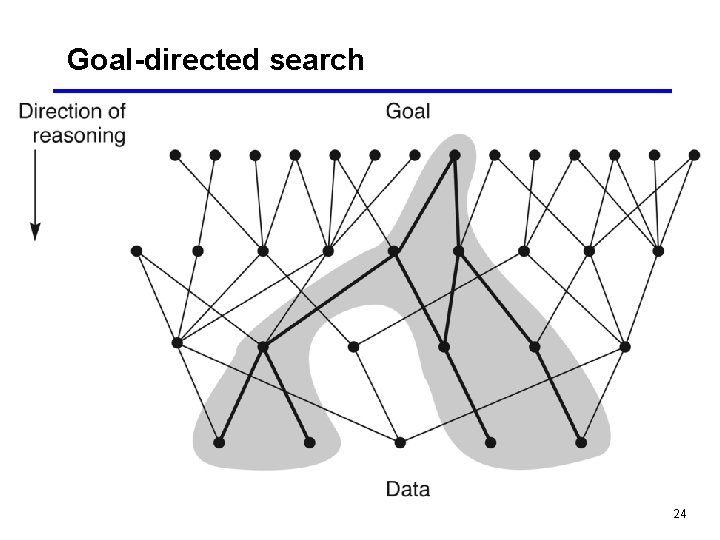Goal-directed search 24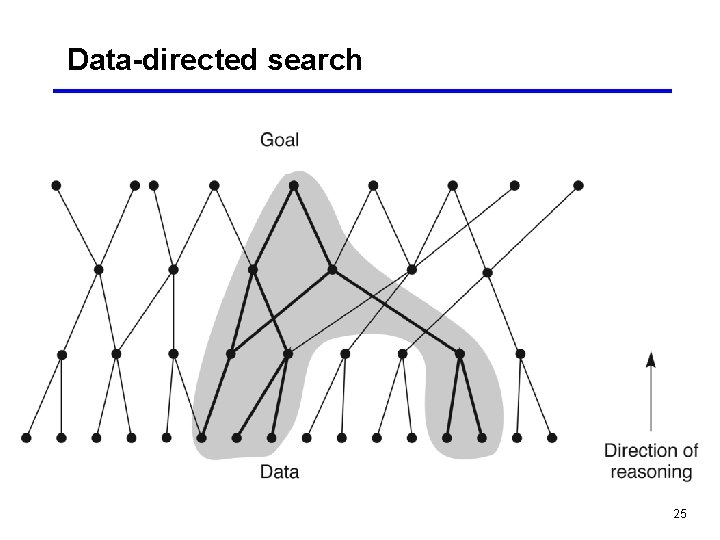Data-directed search 25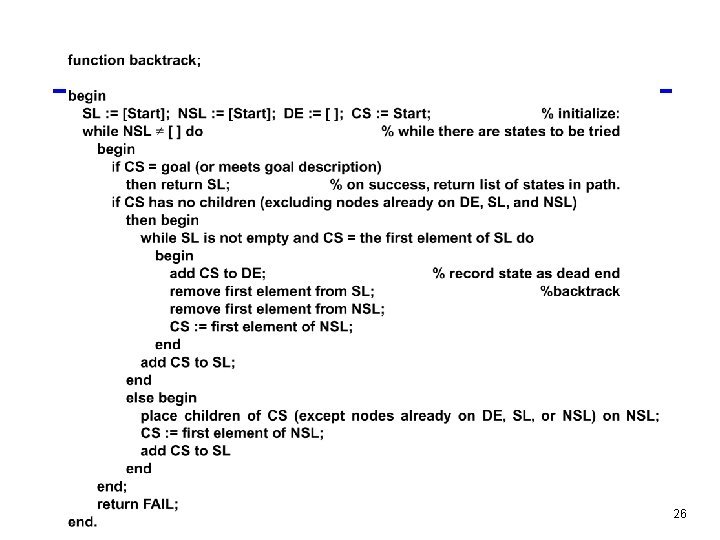26Trace of backtracking search (Fig. 3. 12) 27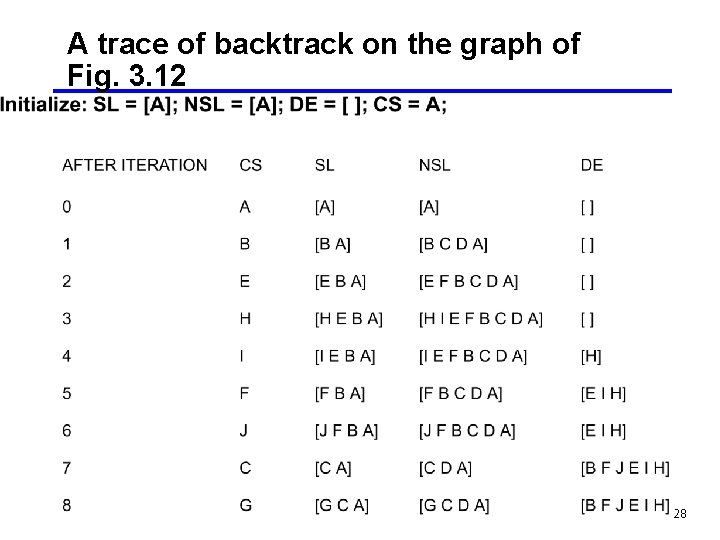A trace of backtrack on the graph of Fig. 3. 12 28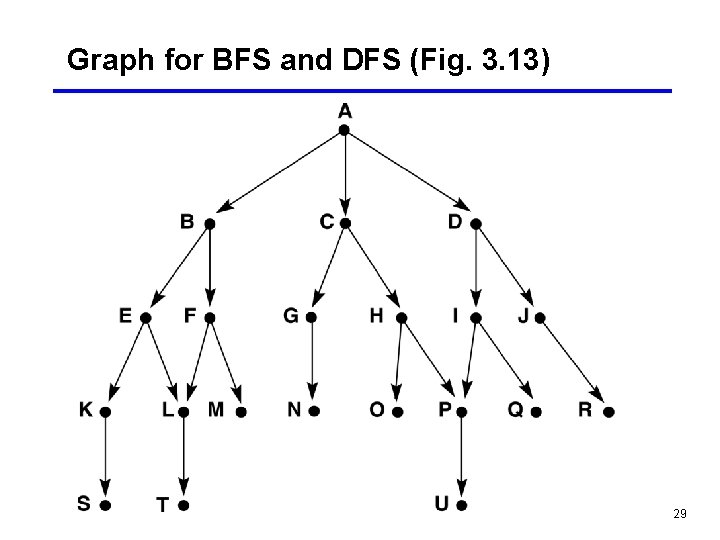Graph for BFS and DFS (Fig. 3. 13) 29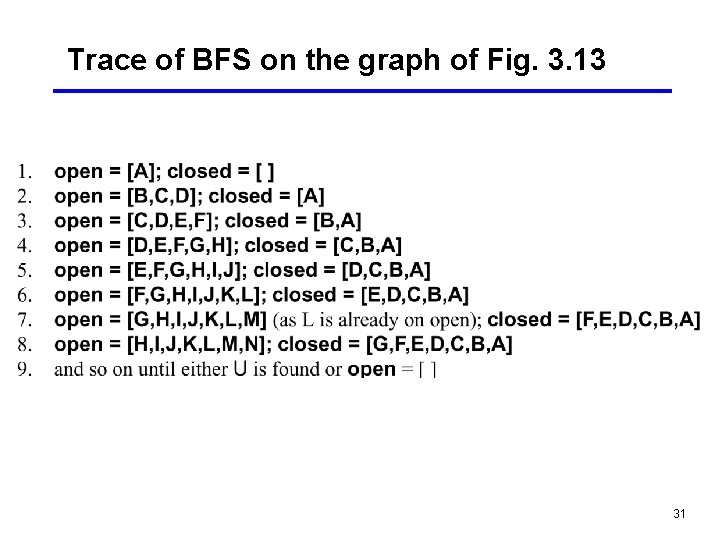Trace of BFS on the graph of Fig. 3. 13 31Graph of Fig. 3. 13 at iteration 6 of BFS 32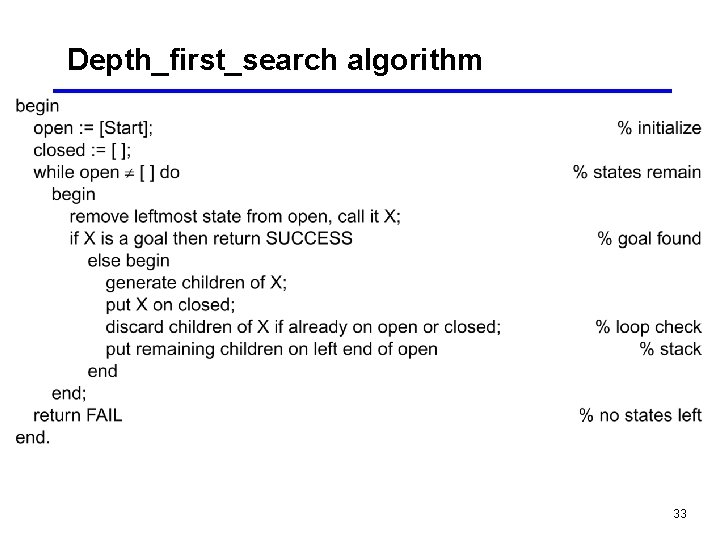Depth_first_search algorithm 33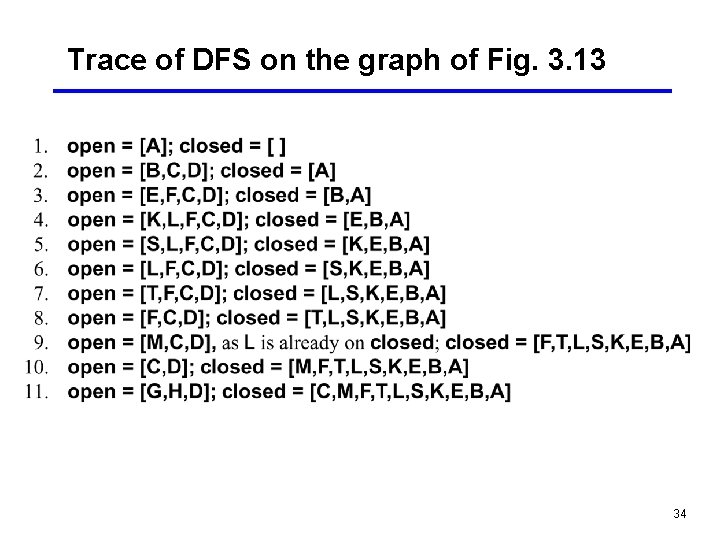Trace of DFS on the graph of Fig. 3. 13 34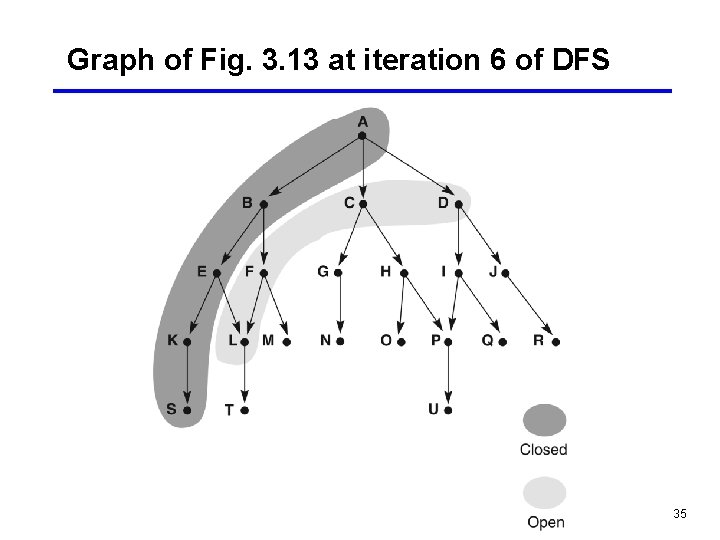Graph of Fig. 3. 13 at iteration 6 of DFS 35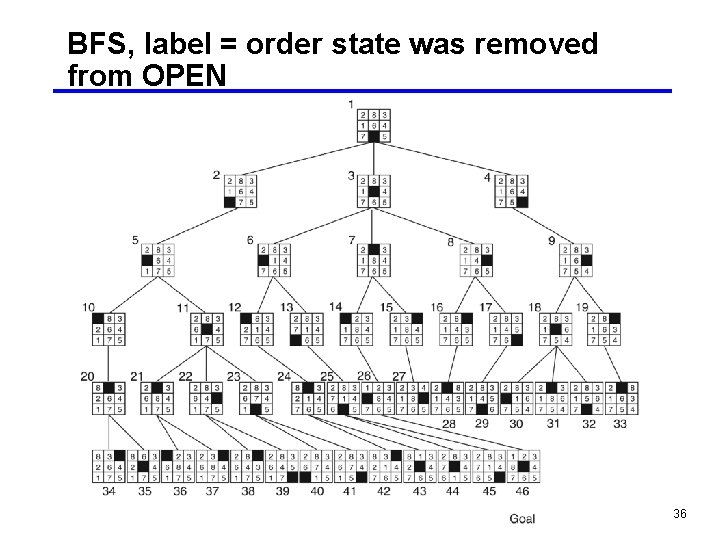BFS, label = order state was removed from OPEN 36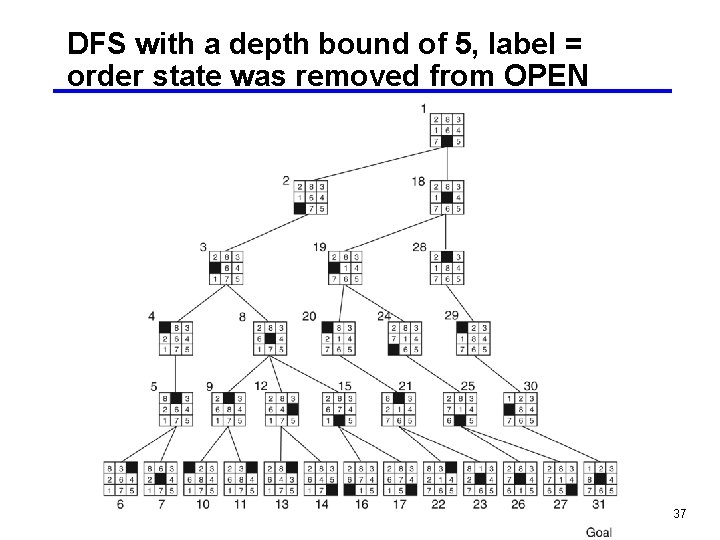DFS with a depth bound of 5, label = order state was removed from OPEN 37State space graph of a set of implications in propositional calculus 38And/or graph of the expression q r p 39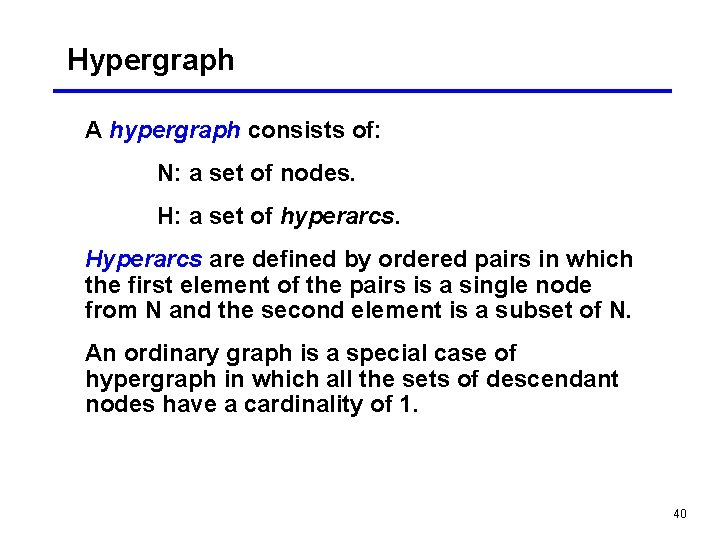Hypergraph A hypergraph consists of: N: a set of nodes. H: a set of hyperarcs. Hyperarcs are defined by ordered pairs in which the first element of the pairs is a single node from N and the second element is a subset of N. An ordinary graph is a special case of hypergraph in which all the sets of descendant nodes have a cardinality of 1. 40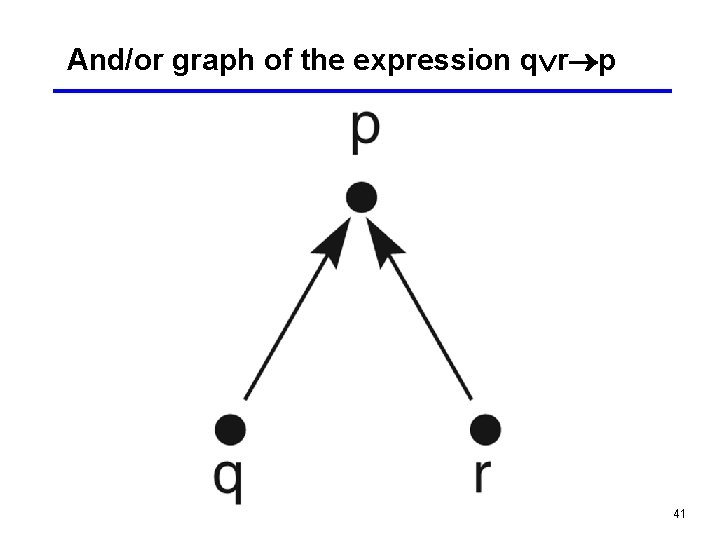And/or graph of the expression q r p 41And/or graph of a set of propositional calculus expressions 42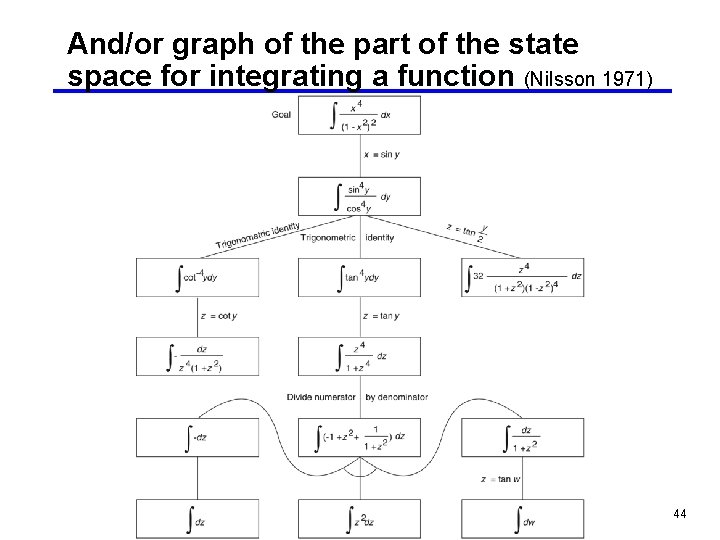And/or graph of the part of the state space for integrating a function (Nilsson 1971) 44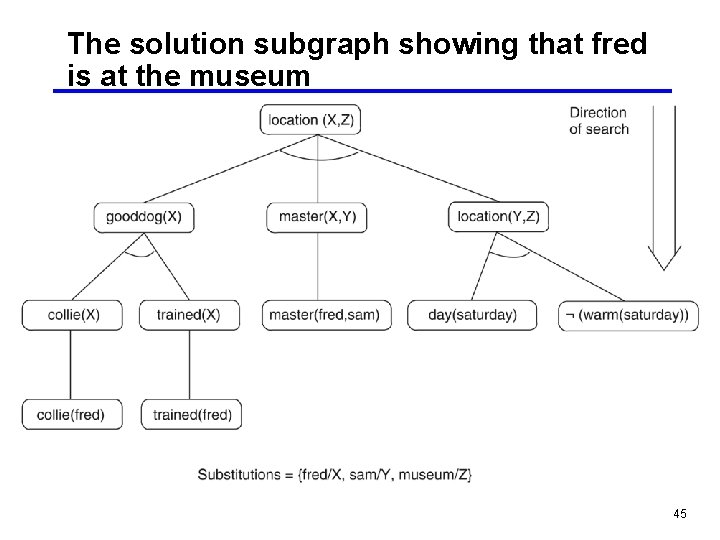The solution subgraph showing that fred is at the museum 45Facts and rules for the example 46Five rules for a simple subset of English grammar 47Figure 3. 25: And/or graph for the grammar of Example 3. 3. 6. Some of the nodes (np, art, etc. ) have been written more than once to simplify drawing the graph. 48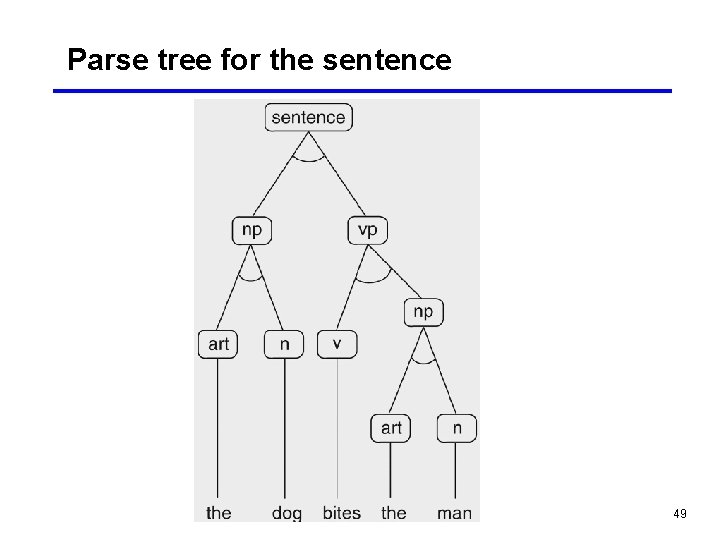Parse tree for the sentence 49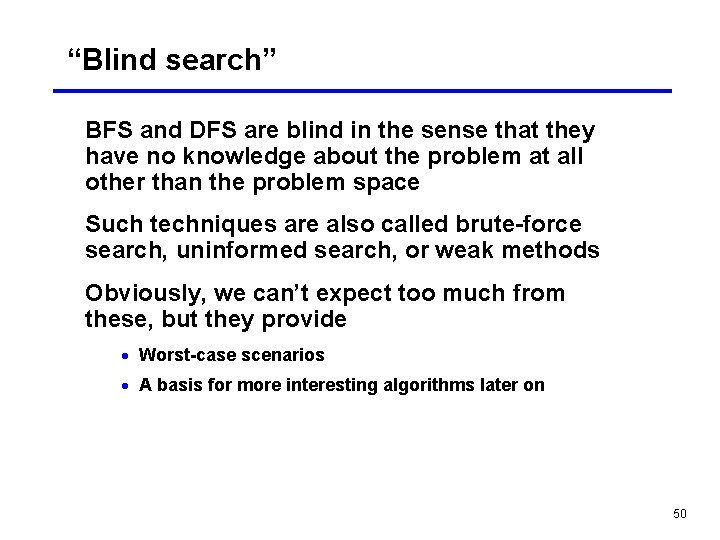“Blind search” BFS and DFS are blind in the sense that they have no knowledge about the problem at all other than the problem space Such techniques are also called brute-force search, uninformed search, or weak methods Obviously, we can’t expect too much from these, but they provide · Worst-case scenarios · A basis for more interesting algorithms later on 50BFS and DFS Worst case scenarios are equally bad (exponential) How to evaluate search algorithms · Completeness: a search algorithm is complete if it is guaranteed to find a solution when one exists · Quality of solution: usually the path cost · Time cost of finding the solution: usually in terms of number of nodes generated or examined · Memory cost of finding the solution: how many nodes do we have to keep around during the search 51Evaluating BFS • Complete? Yes • Optimal quality solution? Yes • Time required in the worst case O(bd) • Memory required in the worst case (in OPEN) O(bd) where b is the branching factor, d is the depth of the solution 52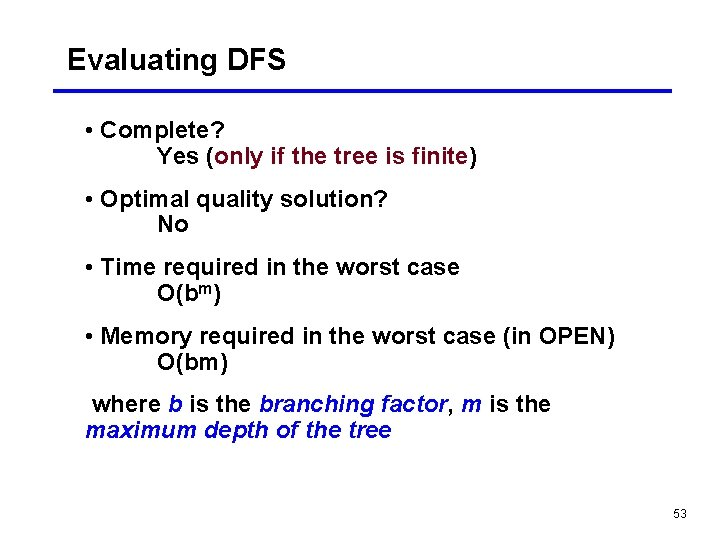Evaluating DFS • Complete? Yes (only if the tree is finite) • Optimal quality solution? No • Time required in the worst case O(bm) • Memory required in the worst case (in OPEN) O(bm) where b is the branching factor, m is the maximum depth of the tree 53Example search problems Puzzles: missionaries and cannibals, cryptarithmetic, 8 -puzzle, 15 -puzzle, Rubik’s cube, n-queens, the Tower of Hanoi, … 2 -player games: chess, checkers, Chinese Go, … Proving theorems in logic and geometry Path finding “Industrial” problems: VLSI layout design, assembling a complex object “AI problems”: speech recognition, planning, … 54The importance of the problem space The choice of a problem space makes a big difference in fact, finding a good abstraction is half of the problem Intelligence is needed to figure out what problem space to use still poorly understood: the human problem solver is conducting a search in the space of problem spaces 55